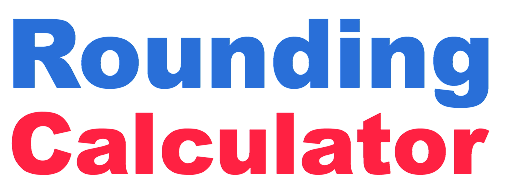Rounding CalculatorOur rounding calculator rounds any number according to how accurate you want it. To get started, please enter your number below and how accurate you want the answer to be.    Ones Tenths Hundredths Thousandths Ten Thousandths Hundred Thousandths Millionths Ten Millionths Hundred Millionths Billionths How does the Rounding Calculator work? We look at how accurate and how many decimals you want, based on our Rounding Table. Then we look at the number to the right of the last decimal you want. If that number is 5 or more, then we will increase your last decimal by 1. If it is 4 or less, your last decimal stays the same. If the number you entered has less decimals than the number of decimals you want, then we simply add 0s to your number.

 Rounding Table What do the different rounding accuracy options mean? Ones = 0 decimals (integer) Tenths = 1 decimal Hundredths = 2 decimals Thousandths = 3 decimals Ten Thousandths = 4 decimals Hundred Thousandths = 5 decimals Millionths = 6 decimals Ten Millionths = 7 decimals Hundred Millionths = 8 decimals Billionths = 9 decimals